The Art of Code is a MA/MFA class that I am teaching at Multidisciplinary New Media department in Paris College of Art. The course provides an introduction to coding for artists and designers who are willing to learn and use it as a form of creative expression.

This website documents
the course materials. If you have any questions, you can contact me on Twitter.

# Basics

p5.js has specific structures, conventions in place to help start coding and getting results quickly. Many functions and variables are already defined so we can call them. At the same time, there are functions called by p5.js when needed, and we define what they should do.

These are few functions that p5.js calls when needed. We define what they should do:

• `setup()`
• `draw()`
• `mousePressed()`
• `preload()`

There are variables defined by p5.js that we can use:

• `windowWidth`
• `windowHeight`
• `width` (only after canvas is created)
• `height` (only after canvas is created)
• `frameCount`
• `mouseX`
• `mouseY`
• `deviceOrientation` (if available)

And there are functions defined by p5.js that we can use:

• `createCanvas()`
• `rect()`
• `circle()`
• `line()`
• `fill()`
• `stroke()`
• and many more...

Full documentation is on the p5.js website.

A basic p5.js sketch contains a `setup()` and a `draw()` function.

``````

// Global variables go here if needed

function setup() {
// Setup function is run once the sketch starts

}

function draw() {
// Draw runs on loop, with a framerate after setup is done

}
```
```

## Variables

Variables are containers for storing information to be used and/or manipulated later. They can store different types of data: integer, float, string, etc. We use `let` or `const` to declare them. Variables declared with `let` are mutable (changeable). Variables declared with `const` are read-only, and they cannot be changed once declared (immutable).

``````
// An integer
let myVariable = 450;

// A float
let mySecondVariable = 430.5;

// A String
let myText = 'Hello, how are you?';

// Immutable variables
const primaryNumber = 45;
const secondNumber = 28.3;
const thirdName = 'John';
```
```

## Arrays

Array is a list-like object (data structure) that contains other elements. In JavaScript, neither the length nor the types of its elements are fixed. An array's length can change at any time. Arrays use integers as element indexes. Adopted from MDN web docs

How to define arrays?

``````
// An empty array
let sampleList = [];

// Array with number
let randomNumbers = [3, 5, 6, 8, 10, 45, 567];

// Array with strings
let names = ["Sophia", "Charlotte", "William", "Oliver"];

// Mixed array
let everything = [6, "Sophia", 475, 67, 32, 4, "Oliver", 837];
```
```

How to modify arrays? Add, remove elements...

``````
let arr = [];

// Print the length of the array
print(arr.length);

// Add an element
arr.push(3);

// Remove last element
arr.pop();

// Remove first element
arr.shift();
```
```

## Functions

"...a sequence of program instructions that performs a specific task"Wikipedia

p5.js comes with a lot of pre-defined functions that you can use to execute specific tasks (draw a shape, show an image, play audio...) You can also define your functions to make it easy to perform repetitive tasks.

``````
// Define a function named myFunction
function myFunction() {
// Instructions to be executed when function is called
// goes inside curly braces
}

// Define setup() and call our function inside
function setup() {
myFunction();
}
```
```

Optionally, functions can have parameters. Parameters are variables that are scoped to the function, that can be assigned a value when calling the function. — p5.js docs

``````
function drawYellowCircle(x, y, size) {
fill(255, 255, 0);
circle(x, y, size);
}

drawYellowCircle(5, 10, 30);
```
```

Functions can also return a value:

``````
// It is not useful to write a function
// for a basic arithmetic operation
// but it works well as a simple example :)

function add(value1, value2) {
return value1 + value2;
}

let result = add(3, 9);

print(result);
// prints 12 to the console.
```
```

## Iteration loops

Iteration loops are structures that execute specified tasks until a particular condition is met.

``````

for (let i = 0; i < 50; i++) {
// Tasks inside curly braces are repeated 50 times
}

// We can use variables to define total amount
let total = 100;
for (let i = 0; i < total; i++) {
// Tasks inside are repeated 100 times
}

// Example
// Numbers from 0 to 9 will be printed to the console.
for(let i = 0; i < 10; i++){
print(i);
}
```
```

How to iterate through an array?

``````
let someNumbers = [3, 5, 6, 8, 10, 45, 567];

for(let i = 0; i < someNumbers.length; i++){
print(someNumbers[i]);
}
// Numbers in the array will be printed to the console.
```
```

While loop is another way of iteration:

``````
// Code inside while block will be executed
// as long as the condition is true.
// If condition is always true, it will repeat
// infinitely, crashing your browser tab.

let v = 10;
while(v < 20) {
print(v);
v++;
}
```
```

## Exercises

Try to replicate shapes below with code. These functions might help you to start: `background()` `circle()` `fill()` `stroke()` `strokeWeight()` `line()` `rect()`. For more information, have a look at the official documentation.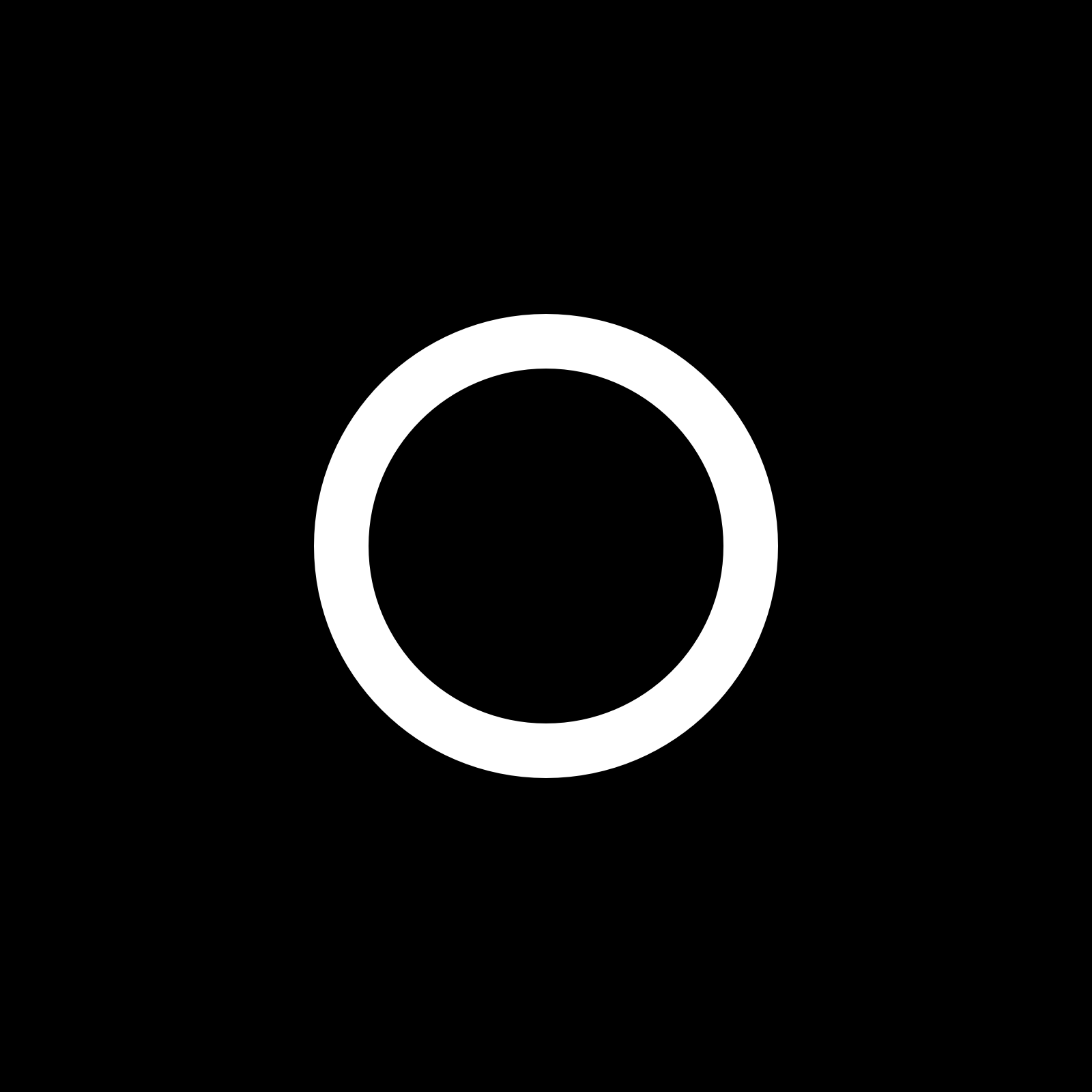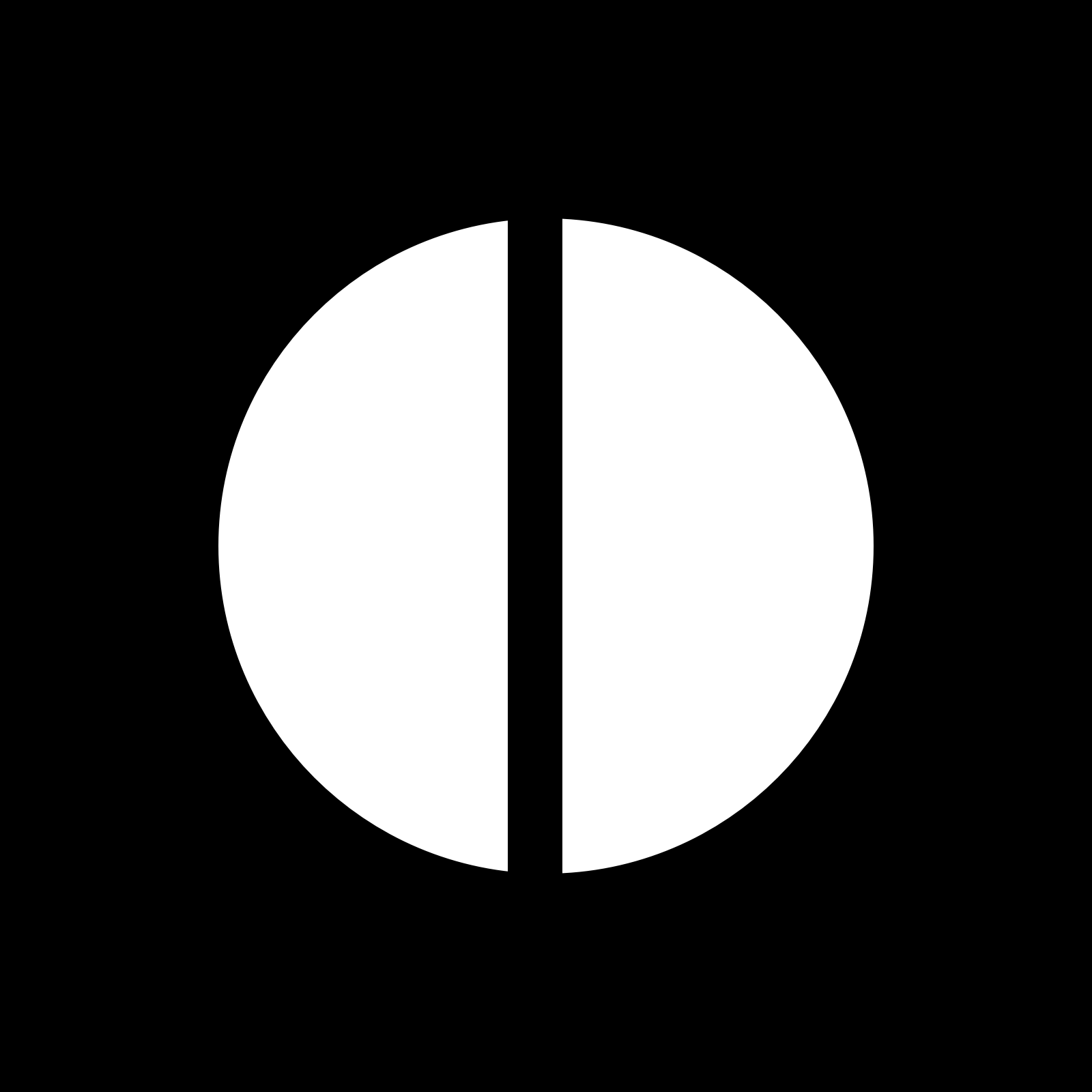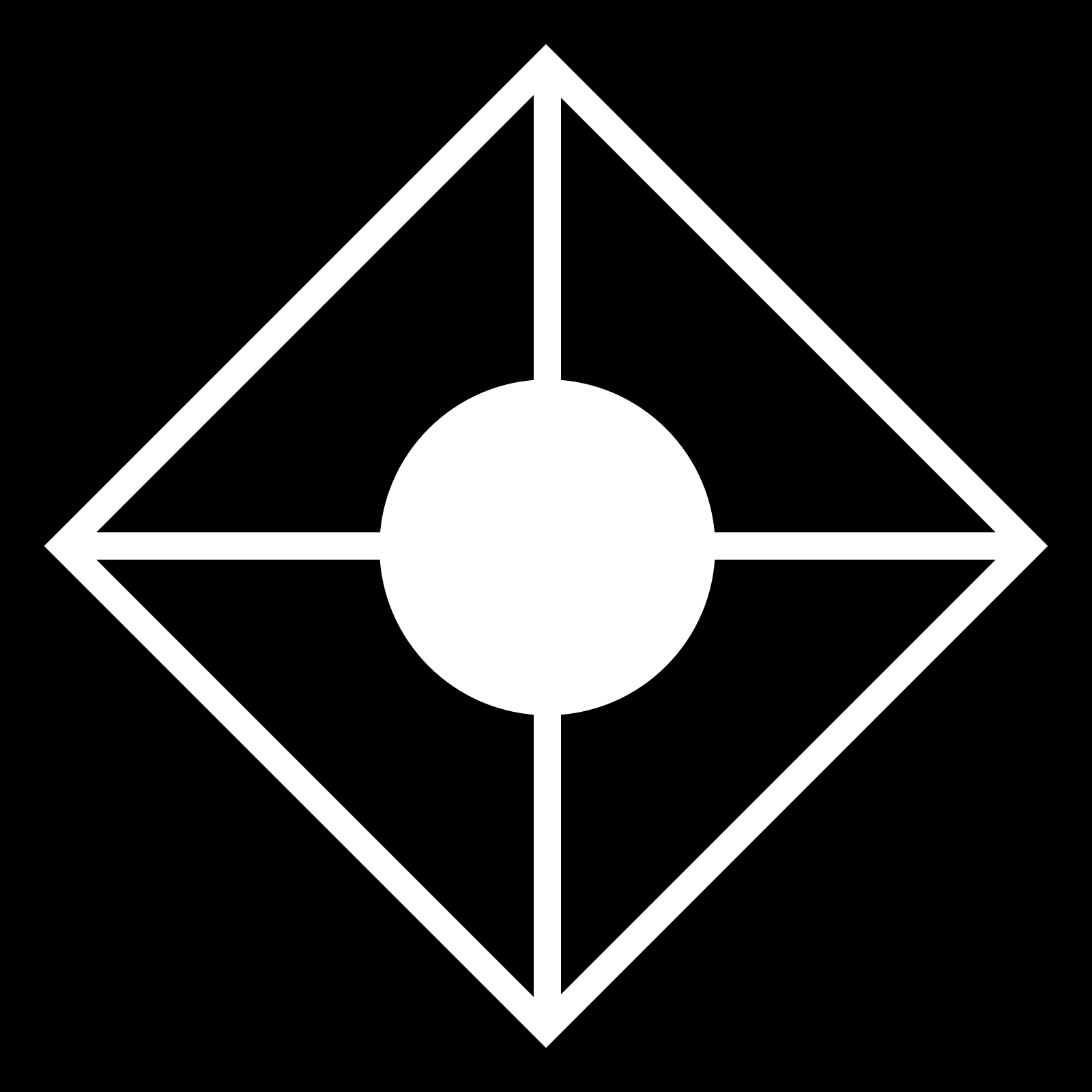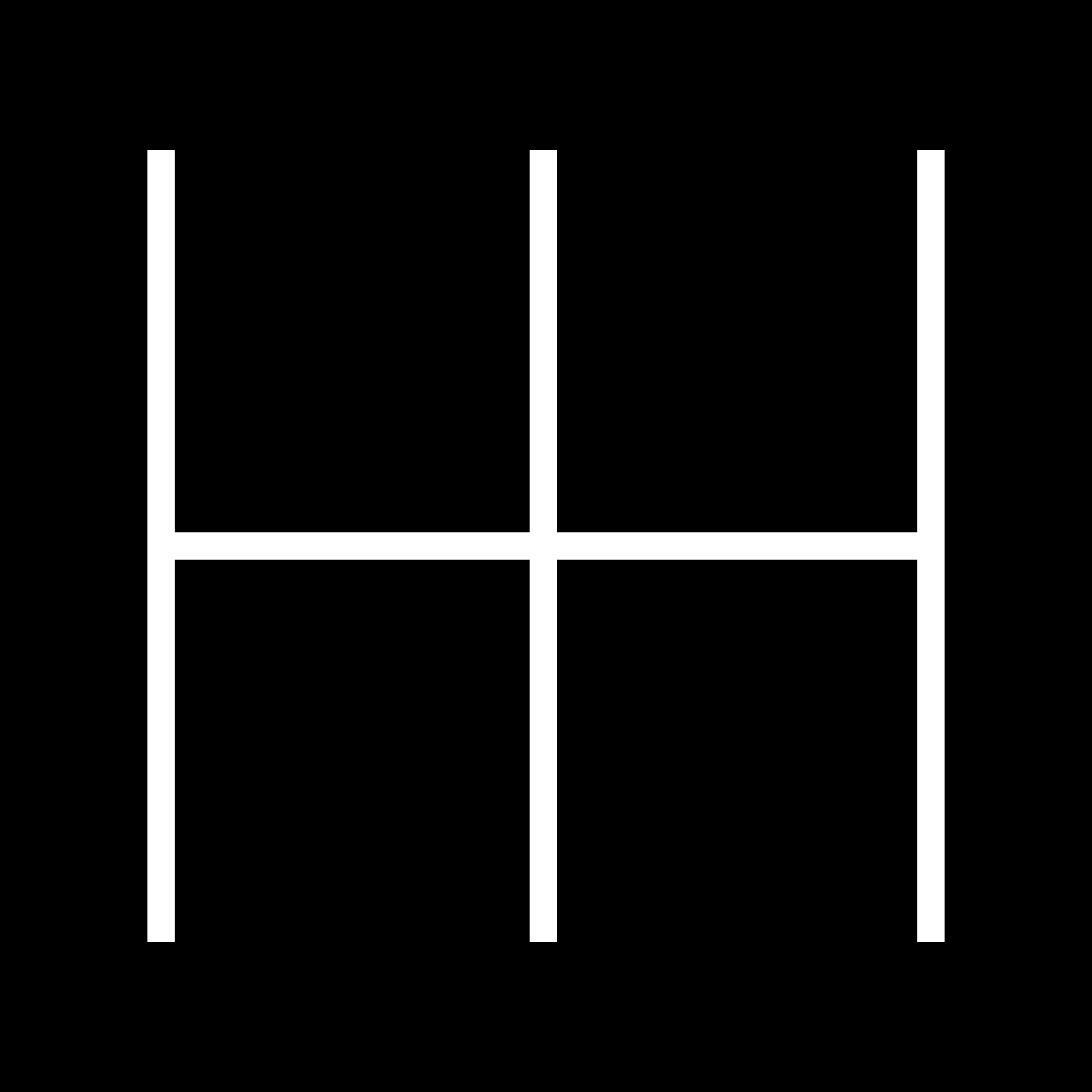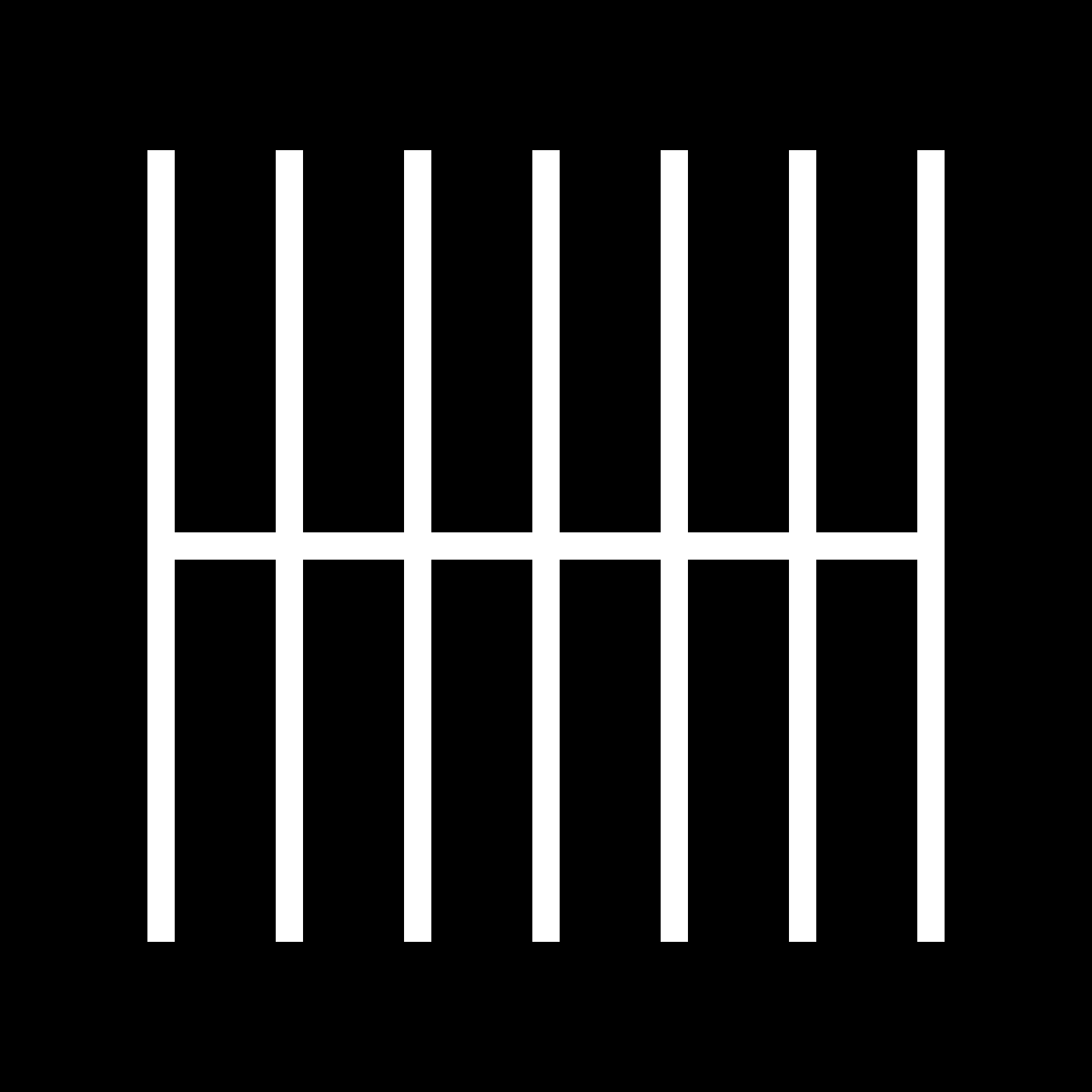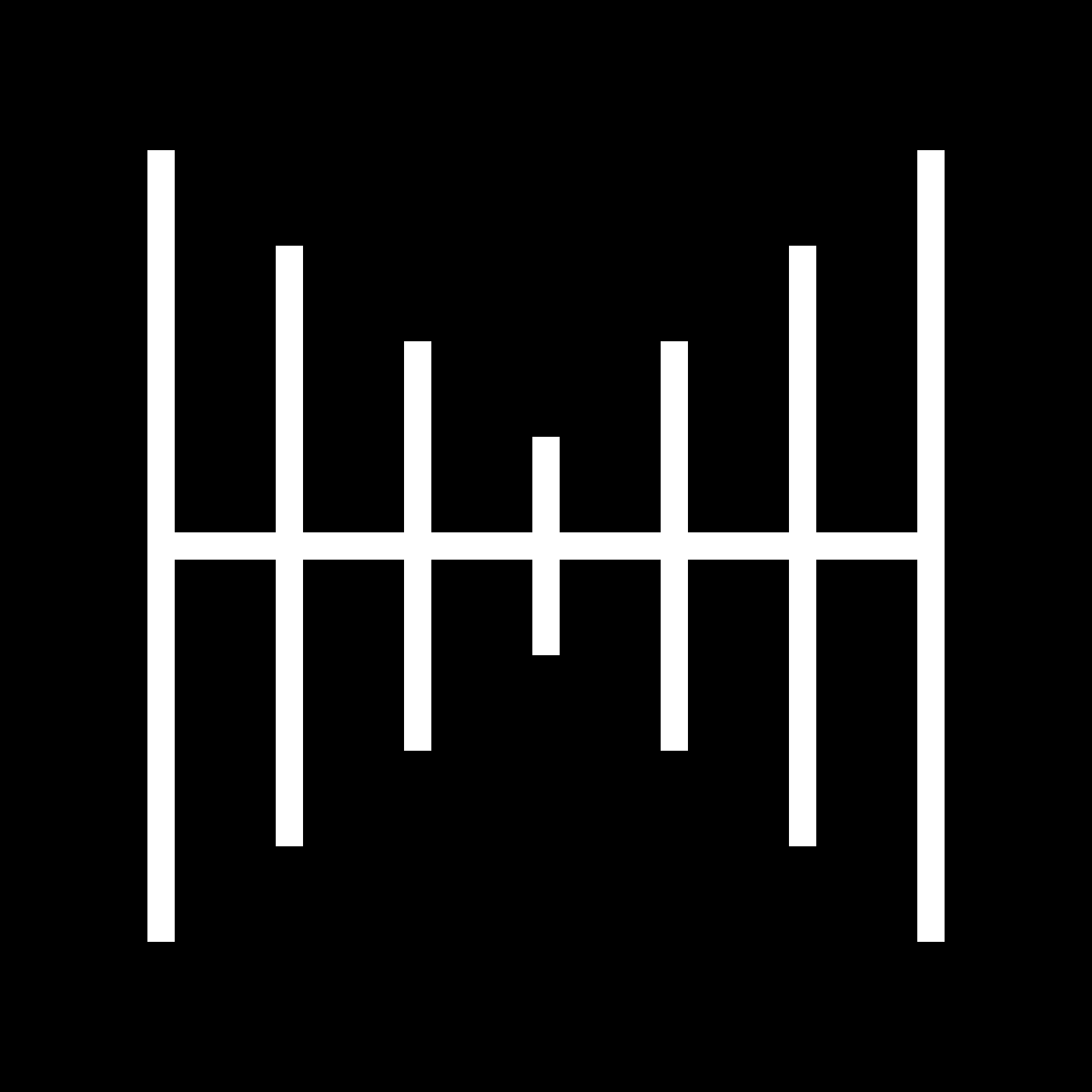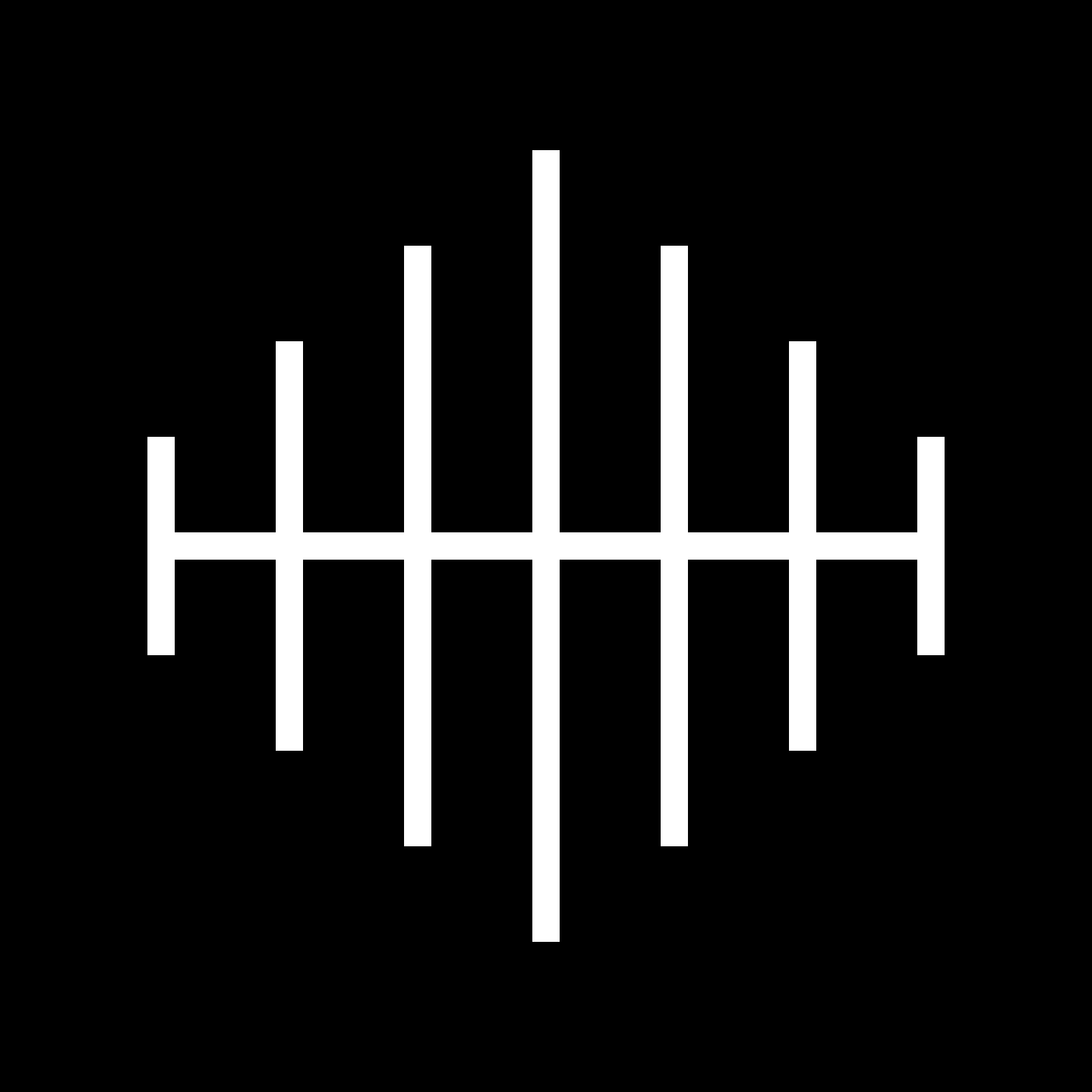## Read More

Official p5.js Reference

The Coding Train - Shapes & Drawing